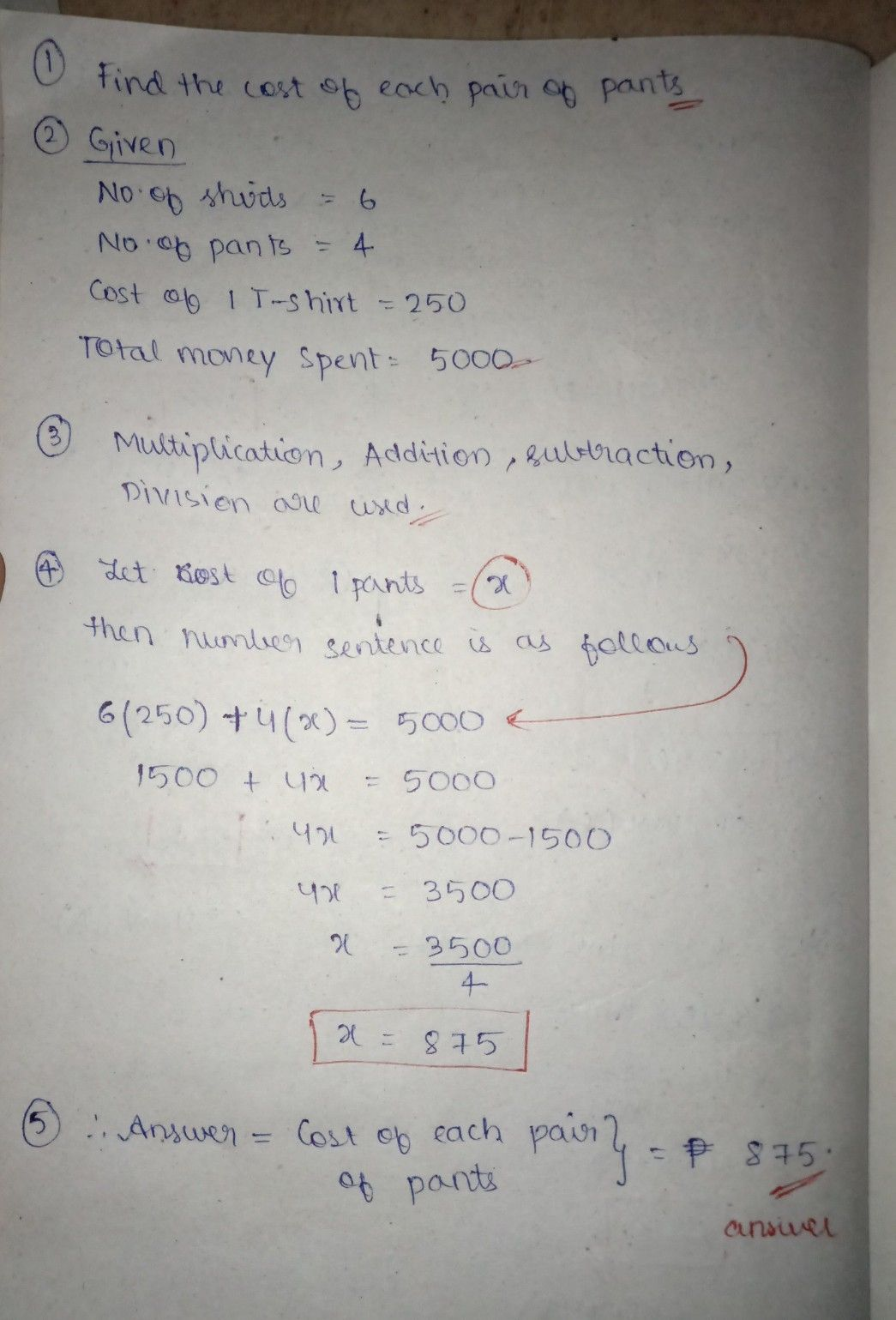Symbol
ProblemProblem Soving NumIbnverols vainng d DMiovinseiy on of Decimal After going Lesson $non-roatinc$ problthermous gh this lesson, involving divisyioon u of are $c\times pcctc9$ to solve routine and whole strategines uambned rs tools. including money using decimals, mixed decimals, and appropriate problem solving $Again$ one thing that you have to consider in solving routine and solve the first non-riot uftior ne problems, are the different steps in solving it. If you failed to ife won't $stoP$ there time, don't if you failed worry. There is always a $sccon4$ time. Your to do most of it. $1cstions$ in your notebook. Learning Task 1: Read and analyze the given $prob1cm$ $Ansy4+1$ the given Salima bought 6 $T-sbi\pi s$ and 4 pairs of $Pant$ shirts $cos1$ P $250.o0$ cach, find the cost of each $ts$ $iorP$ $5000.00$ $pairotpant$ If the 1. What is asked? $2.$ $3.$ What are the given? 45. . What is the number $scn1cncc$ What operation will be used? What is the answer $t01bc$ $problcm$ D
1st-6th grade
Algebra
Search count: 111
SolutionQanda teacher - Kavitha
thank you for asking PREFERRED MATCH dear ?
apologies for the delayIf you have any doubts please do let me know dear. If u want solutions of any other questions , ask me in preferred match ques .. if you are satisfied with my answer please .. do LIKE and give a 5 STAR RATING !!. ALL THE BEST..!!!